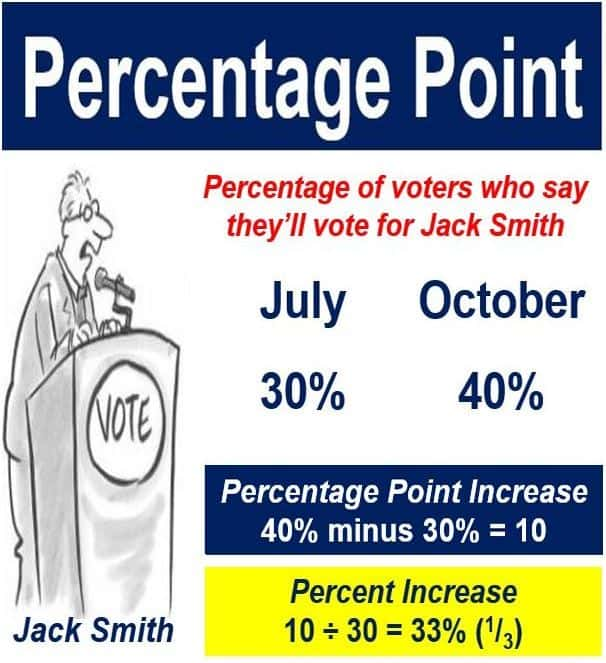What is a percentage point? Definition and meaning

A percentage point, also known as a percent point or pp, is a one-hundredth. If something increases by one percentage point it does not mean it increased by 1% – people commonly confuse the two, but they are quite different.

Percentage point refers to the arithmetical difference between two percentages. 10% is one percentage point more than 9%.

If a country’s GDP (gross domestic product) grew by 2% in 2016 and by 1% in 2015, it means that GDP in 2016 was one percentage point higher than in 2015, but not one percent (1%) higher – it was 100% higher (2% is double 1%). When something is 100% greater, it means it is double.The percentage point difference is simply the arithmetical difference between the two numbers, which in this case is +10. For percentage difference: divide the difference between the two numbers (10) by the first number (30), which in this case gives you +33% or one third.

Five percent compared to four percent, four percent versus three percent, 3% contrasted with 2% – all have a difference of one percentage point, but in the first case 5% is 25% greater than 4%, in the second case four percent is 33% greater than three percent, and in the last case 3% is 50% greater than 2%.

According to MathsisFun.com, there are…:

“…two correct ways to talk about a rise from 10% to 12%: A. A rise of 20%. B. A rise of 2 percentage points. When in doubt, use both. For example, ‘Interest rates increased by 2 Percentage Points today, meaning a 20% increase in interest payments.'”

Percentage point – proportion of smokers

Imagine you carried out a study on the proportion of people who smoked in your country over time – from 1996 to 2016.

• In 1996, the proportion of the adult population who were regular smokers stood at 40%.
• In 2016, the percentage dropped to 30%.

Over that twenty-year period, the prevalence of regular smoking among adults declined by:

• 10 percentage points; the arithmetical difference between 40 and 30 is ten.
• 25%; smoking prevalence fell by one quarter. Forty minus one quarter or 25% is thirty.If your are writing an article, be extra careful when using the terms percent or percentage point.

Percentage point – risk or probability

Percentage point differences are commonly used when we are talking about probability or risk.

Imagine there is a new drug – called Cureitall – that cures 75% of all patients with a fatal disease, compared to just 50% of patients on other treatments.

By taking this new drug, the absolute risk of dying from the disease is reduced by 25 percentage points.

Consumers of statistics say NNT (number needed to treat) is more meaningful. The reciprocal transform of the percentage point difference would be 1 ÷ (25%) = 1 ÷ 0.25 = 4.

Thus, if 4 patients are treated with Cureitall, you could expect to cure one more case of the disease than would have happened in its absence.

You could also say that patients’ survival rates improve by 50% if they are administered Cureitall – 50% = half; half of 50 is 25; twenty-five plus fifty is 75.

Video – percentage point vs. percent

This video explains the definition and meaning, using easy-to-understand terms and examples, what the difference between a percentage point and percent change is. The speaker uses a politician’s change in popularity as an example.